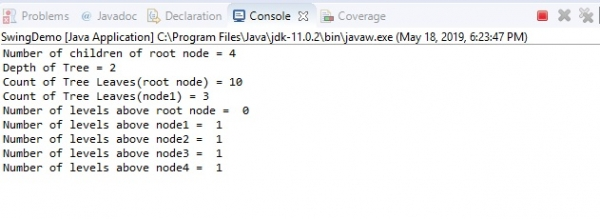# Get the number of levels above a node in JTree with Java

To get the number of levels above a node, use the getLevel() method. Following is an example for the root node “node” −

node.getLevel()

Note − The value 0 is returned if the node is a root node since there are zero levels above the root node.

For other nodes, get the number of levels above a node as shown below with node3 −

node3.getLevel()

The following is an example to get the number of levels above a node in a JTree −

package my;
import javax.swing.JFrame;
import javax.swing.JTree;
import javax.swing.tree.DefaultMutableTreeNode;
public class SwingDemo {
public static void main(String[] args) throws Exception {
JFrame frame = new JFrame("Demo");
DefaultMutableTreeNode node = new DefaultMutableTreeNode("Project");
DefaultMutableTreeNode node1 = new DefaultMutableTreeNode("Domain");
DefaultMutableTreeNode node2 = new DefaultMutableTreeNode("Topics");
DefaultMutableTreeNode node3 = new DefaultMutableTreeNode("QA");
DefaultMutableTreeNode node4 = new DefaultMutableTreeNode("Tools");
DefaultMutableTreeNode one = new DefaultMutableTreeNode("Management ");
DefaultMutableTreeNode two = new DefaultMutableTreeNode("Finance");
DefaultMutableTreeNode three = new DefaultMutableTreeNode("Networking");
DefaultMutableTreeNode four = new DefaultMutableTreeNode("MIS");
DefaultMutableTreeNode five = new DefaultMutableTreeNode("DBMS");
DefaultMutableTreeNode six = new DefaultMutableTreeNode("CSS");
DefaultMutableTreeNode seven = new DefaultMutableTreeNode("MongoDB");
DefaultMutableTreeNode eight = new DefaultMutableTreeNode("Python QA");
DefaultMutableTreeNode nine = new DefaultMutableTreeNode("jQuery QA");
DefaultMutableTreeNode ten = new DefaultMutableTreeNode("Code Formatter Tool");
JTree tree = new JTree(node);
for (int i = 0; i < tree.getRowCount(); i++) {
tree.expandRow(i);
}
tree.putClientProperty("JTree.lineStyle", "Angled");
System.out.println("Number of children of root node = " + node.getChildCount());
System.out.println("Depth of Tree = " + node.getDepth());
System.out.println("Count of Tree Leaves(root node) = " + node.getLeafCount());
System.out.println("Count of Tree Leaves(node1) = " + node1.getLeafCount());
System.out.println("Number of levels above root node = " + node.getLevel());
System.out.println("Number of levels above node1 = " + node1.getLevel());
System.out.println("Number of levels above node2 = " + node2.getLevel());
System.out.println("Number of levels above node3 = " + node3.getLevel());
System.out.println("Number of levels above node4 = " + node4.getLevel());
tree.setRowHeight(20);
frame.setSize(600,450);
frame.setVisible(true);
}
}

The following is the JTree −## Output